# 5 Probability Distributions

“Statistics is the grammar of science.”

— Karl Pearson

Learning Objectives
1. Learn the definitions of random variables, probability distributions, and expected values and the connections between them.
2. Understand how the parameters of a distribution govern the shape of the distribution
3. Relate probability distributions to data generated according to a distribution
4. Learn about the binomial and normal distributions, their parameters, and how their distribution shape is related to those parameters.

## 5.1 Introduction to Probability Distributions

In Chapter 4, we introduced the idea of random processes, i.e. situations in which the outcome can not be determined perfectly in advance. Random processes are defined in terms of the collection of possible events (sample space) and their associated probabilities. In that chapter, we saw three methods for calculating probabilities – the enumeration method, the probability function method, and the simulation method. In this chapter we will expand on the probability function method, which uses a known function called a probability distribution to determine the probability of each event.

Probability distributions are closely related to random variables, numeric variables that can take on different values depending on the outcome of a random process. In previous mathematics courses you may have seen variables such as $$x$$ or $$y$$ used as placeholder values which are then solved for. For example, you can solve for the variable $$x$$ in $$4x + 5 = 25$$, to determine $$x = 5$$. By contrast, the outcome of a random variable is not be predetermined. Instead, we talk probabilistically about the likelihood of observing each possible outcome. Random variables are typically denoted with capital letters, e.g., $$X$$ or $$Y$$, whereas the observed outcome of the random process is denoted with lowercase letters $$x$$ or $$y$$. For example, flipping a coin three times is a random process. We can define the random variable $$X$$ to represent the number of heads we observe between the three flips. $$X$$ can take on four possible values: 0, 1, 2, or 3. If we observe 2 heads, we have $$x = 2$$.

Most simply, a probability distribution (often just called a distribution) is a method for taking a possible event as input and giving us the corresponding probability as output; the corresponding probability tells us how likely it is that the specific event will occur, out of all of the possible events. We often say random variables have or follow a probability distribution, as the distribution quantifies the probability of observing the possible values a random variable can take on. We can denote a probability distribution as $$P(X = x)$$, or the probability that the random variable, $$X$$, takes on a generic value, $$x$$.

There are many useful probability distributions that have been defined by mathematicians and statisticians to describe a variety of scenarios:

• Counting the number of successes in a fixed number of trials that can results in either success or failure, such as counting the number of heads when flipping a coin three times

• Counting the number of successes before the first failure in a series of success/failure trials, such as counting the number of days before a light bulb burns out

• Describing the length of time between events that occur at a constant rate, such as the time between phone calls at a help desk

• Describing range of continues values, such as the blood pressure in adults

One can represent a probability distribution visually using a probability histogram. On the x-axis, we have the possible outcomes of the random process – the values the random variable could take. For each outcome, the bar height represents the probability of observing that value. For the coin flipping example, the probabilities of observing each possible number of heads can be represented as: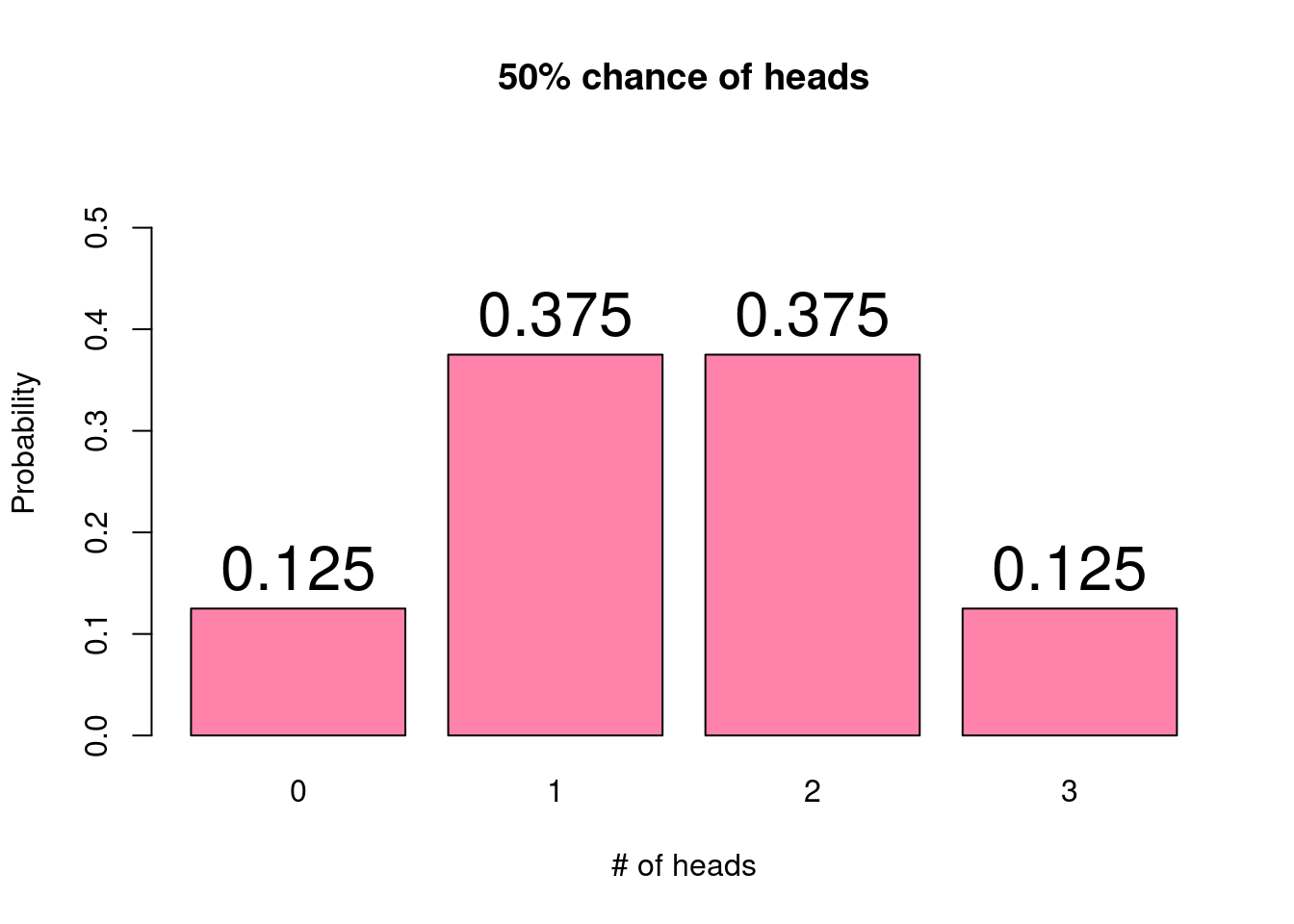When looking at a probability histogram, we can characterize the shape of the distribution analogously to how we talk about a histogram of observed data. We often use unimodal distributions, which may or may not be skewed. For example, the above probability histogram shows a unimodal and symmetric distribution.

The beauty of using probability distributions to describe the likelihood of all outcomes of a random process is its simplicity. Probability distributions rely on a small number of parameters which determine the distribution’s shape. In the coin flipping example, we could consider one of the parameters to be the probability of obtaining heads. We assume that we have a fair coin and that this probability is 50%. If instead we had a weighted coin with a 60% chance of landing on heads, the probability distribution would change. That is, the probability associated with each possible outcome would be different.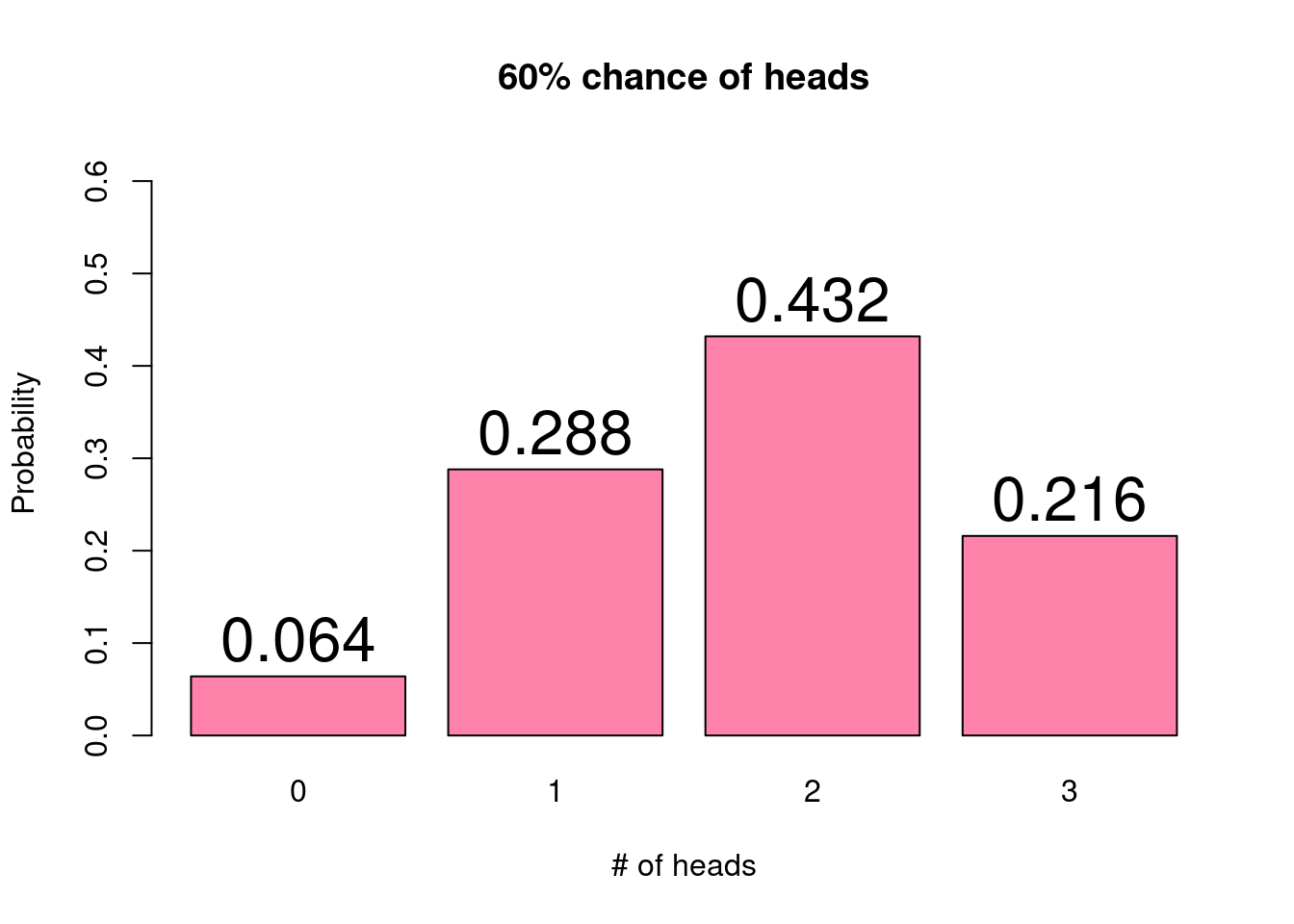With a higher chance of the coin resulting in heads, we now see that the three flips are more likely to result in 2 or 3 heads and much less likely to result in 0 heads. In other words, the distribution is now slightly skewed left. Because these probabilities can be described by a distribution function, we can
easily compute and compare the probabilities of each possible outcome as a function of the distribution parameter, in this case, the probability of flipping heads.

Another key concept related to probability distributions and random variables is the idea of the expected value, often denoted $$E(X)$$. The expected value of a random variable, often denoted as $$E(X)$$, is a weighted average which provides a measure of the central mass of the probability distribution. The expected value averages over all possible outcomes of the random variable with each outcome weighted according to its probability. We can also think of the expected value of a random variable as its average value. In more technical terms, we might say that the expected value is a weighted average which provides a measure of the central mass of the probability distribution, averaging over all possible outcomes of the random variable with each outcome weighted according to its probability. Whew!

Returning to the coin flipping example with a fair coin, we have seen that the probability distribution is:

x P(X = x)
0 0.125
1 0.375
2 0.375
3 0.125

Multiplying each possible outcome ($$x$$) by its associated probability ($$P(X=x)$$) and adding them together (which happens to be the definition of a weighted average) gives us an expected value of

$(0 \times 0.125) + (1 \times 0.375) + (2 \times 0.375) + (3 \times 0.125) = 1.5.$

Looking at the probability histogram, this value should make sense as it falls right in the center of the distribution.

Expected values are more easily conceptualized in terms of a game or bet. For example, consider the following game: you flip a fair coin; if the coin lands on heads, you win $20, and if the coin lands on tails, you lose$1. Would you play this game? Assuming you have a spare dollar, the answer is probably yes. Since you have equal chances of the coin landing on heads or tails, you are just as likely to win $20 as you are to lose$1. In terms of the expected value, it would be

$(20 \times 0.5) + (-1 \times 0.5) = 9.5.$

The expected value tells us that if you were to play this game over and over, you would be expected to win $9.5 per game. Critically, it is worth reiterating here that for any single instance of this game, you can only win$20 or lose $1. The$9.50 indicates the long term average over many games.

Finally, let’s discuss how probability distributions relate back to our general statistical framework. In our framework, we are interested in learning about the population, which we assume follows some probability distribution. This distribution is governed by a set of unknown parameters. When we collect a sample, we expect that the distribution of the sample will be similar to that of the true population. As we will see in the following chapters, our sample will be used to compute statistics, or estimates of the true parameters. Indeed, much of statistical inference involves estimating the values of these parameters, with associated measures of certainty.

We end this chapter with a discussion of two of the most commonly used distributions, the binomial and the normal.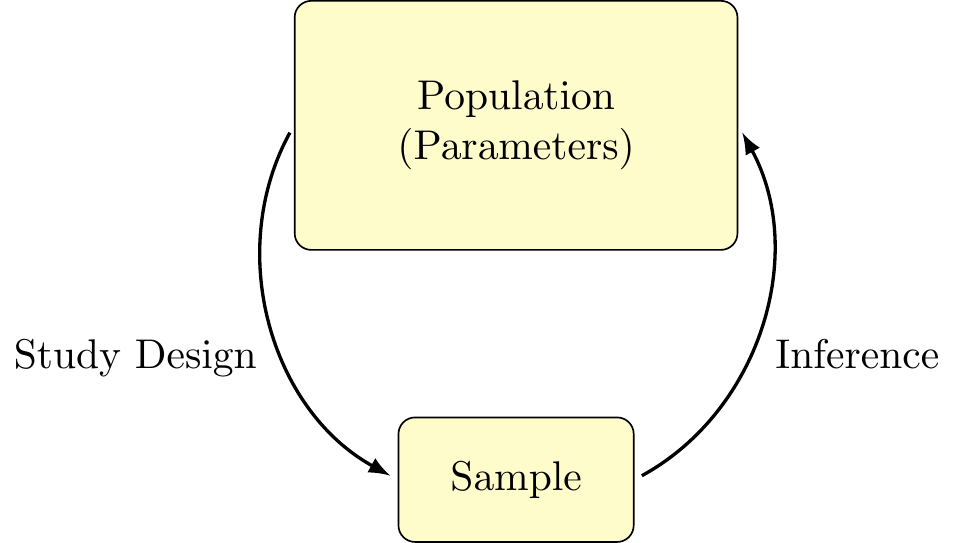Figure 5.1: Statistical Framework (again)

Definition 5.1

Probability distribution: A method for assigning probabilities to all possible events

Random variable: A numeric variable whose value depends on the outcome of a random process

Probability histogram: A graphical display of a probability distribution

Parameters: Values associated with a probability distribution that determine the distributions shape

Expected value: The weighted average of the outcomes of a random variable, with weights determined by their probability

Statistics: Values computed from a sample that serve as estimates of the population parameters

## 5.2 Binomial Distribution

The first distribution we will examine in depth is the binomial distribution, describing the number of successes in a fixed number of independent trials that can result in one of two outcomes (success or failure), where the probability of success is the same between trials. As the data from a binomial distribution fall into distinct categories, the binomial distribution is also a member of a category of distributions known as discrete distributions.

We have already seen one example of the binomial distribution in detail – flipping a coin some number of times and counting the number of flips result in heads (success). Each flip has two possible outcomes (heads or tails), the same probability of heads on each flip (50%), and a predetermined the number of trials (three flips).

To illustrate another example of the binomial distribution, we might consider rolling a six-sided die a number of times, counting the number of rolls that result in either a 5 or 6. That is, we would consider a success to be rolling a 5 or 6, and a failure to be rolling a 1, 2, 3, or 4. As there are six equally likely outcomes, with two of these being considered a success, we note that for any particular roll (trial), the probability of success is 2/6, or 1/3. The takeaway here is that, even though there are six possible values for a given roll, we can still posit a binary outcome and determine the associated probabilities.

As you may have noticed from these two examples, the binomial distribution can be used for various success probabilities and numbers of trials. In fact, these quantities define the two parameters of the binomial distribution. These parameters are typically denoted as $$n$$, the number of trials, and $$p$$, the probability of success. The binomial distribution can be written as a function of these parameters:

$P(X = x) = \binom{n}{x} p^x (1-p)^{n-x}.$

While this may look like a nasty formula, don’t be afraid! Probabilities following a binomial distribution can be easily computed by any statistical software. For this reason, we will focus on the distribution properties rather than performing calculations.

For any valid value of $$n$$ and $$p$$, we can use the binomial distribution to compute the probability of observing any possible outcome. Valid values of $$n$$ and $$p$$ simply mean that the number of trials conducted must be a positive integer (e.g., 1, 2, 3, …) and that $$p$$ must be between 0 and 1 (it is a probability after all). The expected value of the binomial distribution is given by $$E(X) = n \times p$$, or the total number of trials times the probability of success for each trial. To gain a better understanding of how these parameters impact the shape of the distribution, we will use the following applet.

Exercise 5.1

The applet below is designed to help you get familiar with the parameters of the binomial distribution, and how they impact the probability distribution. You can change the values of the number of trials $$n$$, and the probability of success $$p$$, and the app will display the associated distribution in a probability histogram.

Use the applet to answer the following questions:

1. Set $$n = 10$$, and change the value of $$p$$ (note: you can press the triangular “play” button to have the app vary $$p$$ automatically). What happens to the shape of the distribution as $$p$$ gets closer to 0? What about when $$p$$ gets closer to 1?

2. Now, set $$p = 0.4$$ and vary $$n$$ over the range of possible inputs. What do you notice about the x-axis as $$n$$ is changing? Explain this trend by referring back to what $$n$$ represents.

3. Keeping $$p$$ constant and varying $$n$$ between 20 - 50, does the shape of the distribution change? What about the location of the distribution?

Exercise 5.2

The applet below is designed to familiarize you with data generated from the binomial distribution. That is, we are interested in exploring the relationship between an underlying theoretical distribution and data that is observed. You can change the values of the number of trials $$n$$, and the probability of success $$p$$ to specify the parameters of the population distribution. Then, you can take a random sample from the distribution.

Use the applet to answer the following questions:

1. Set $$n = 10$$ and $$p = 0.5$$. Starting with a sample size of 10, simulate data a number of times and observe the histogram of the observed data. What do you notice? Is the observed data typically centered around the same value as the probability distribution? Does it have the same shape? Try again with a sample size of 50. What has changed? What stays the same? Do this once more with a sample size of 100.

2. What are some general observations that can be made from the results in saw in (1)? How consistently does a histogram of the observed data match that from the theoretical distribution? What effect does sample size have on this consistency?

3. Play around with different values of $$n$$ and $$p$$, as well as with different sample sizes. What are some trends that you notice? Are there values of $$n$$ and $$p$$ that seem to produce more consistent results? If you were to only look at the observed data, how confident do you feel that you could guess the shape of the underlying distribution? How close might you be?

4. In general, how would you describe the histogram of the binomial distribution? Does it tend to be symmetric? How do different values of $$p$$ change the skew of the distribution? How about different values of $$n$$?

Definition 5.2

Binomial Distribution: A probability distribution that characterizes the probabilities of observing some number of successes in a fixed number of trials, each with two possible outcomes and the same probability of success

Discrete Distribution: Any probability distribution that depicts the occurrence of distinct, countable values

## 5.3 Normal Distribution

Let’s begin by considering three histograms, each describing a set of continuous data collected from various sources. First, we have the annual depth of Lake Huron from 1875-1972 measured in feet. Next, the annual flow of the river Nile from 1871-1970 measured in cubic meters, and lastly, the recorded height, in feet, of 31 black cherry trees. What do all of these histograms seem to have in common?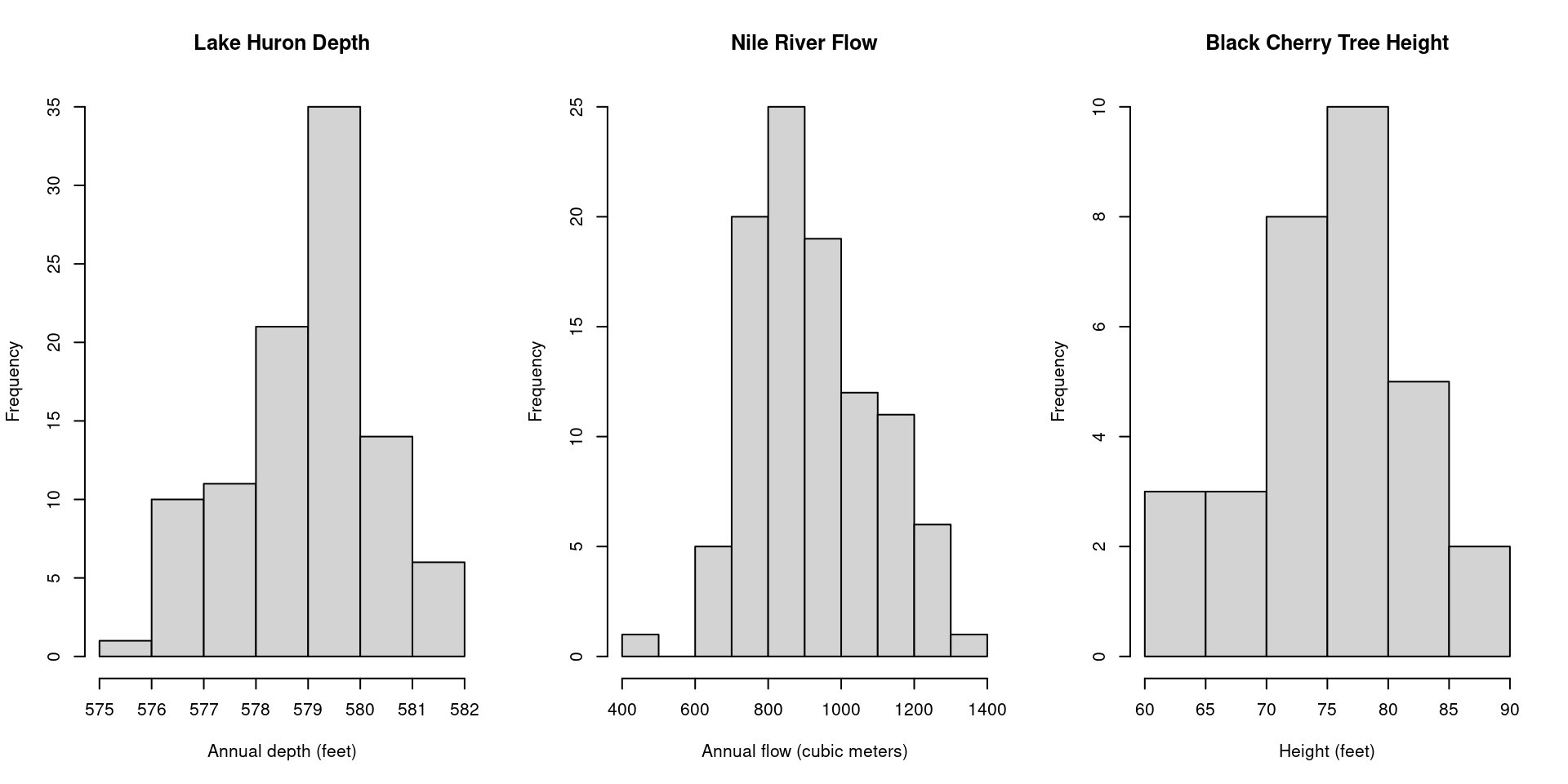What we see here are examples of a normal distribution (also known as a bell curve), one of the most ubiquitous distributions in all of statistics. The normal distribution is characterized by the “bell shape” that is symmetric about it’s center.

Like the binomial, the normal distribution is characterized by two parameters, $$\mu$$ and $$\sigma^2$$, representing the mean and the variance, respectively. The mean value, $$\mu$$, indicates the location of the peak on the x-axis, whereas the variance, $$\sigma^2$$, indicates the amount of dispersion about the mean. A random variable $$X$$ that follows a normal distribution can be expressed $$X \sim N(\mu, \sigma^2)$$, or, “The random variable $$X$$ follows a normal distribution with mean $$\mu$$ and variance $$\sigma^2$$.” The formula for the normal distribution is given as

\begin{align*} f(x) = \frac{1}{\sqrt{2 \pi \sigma^2}} \ e^{- \frac{(x-\mu)^2}{2\sigma^2}}. \end{align*}

Consider the two normal distributions below, with different values for $$\mu$$ and $$\sigma^2$$. Although they are centered at different locations and have different amounts of dispersion around the mean, they are both bell-shaped curves characteristic of the normal distribution: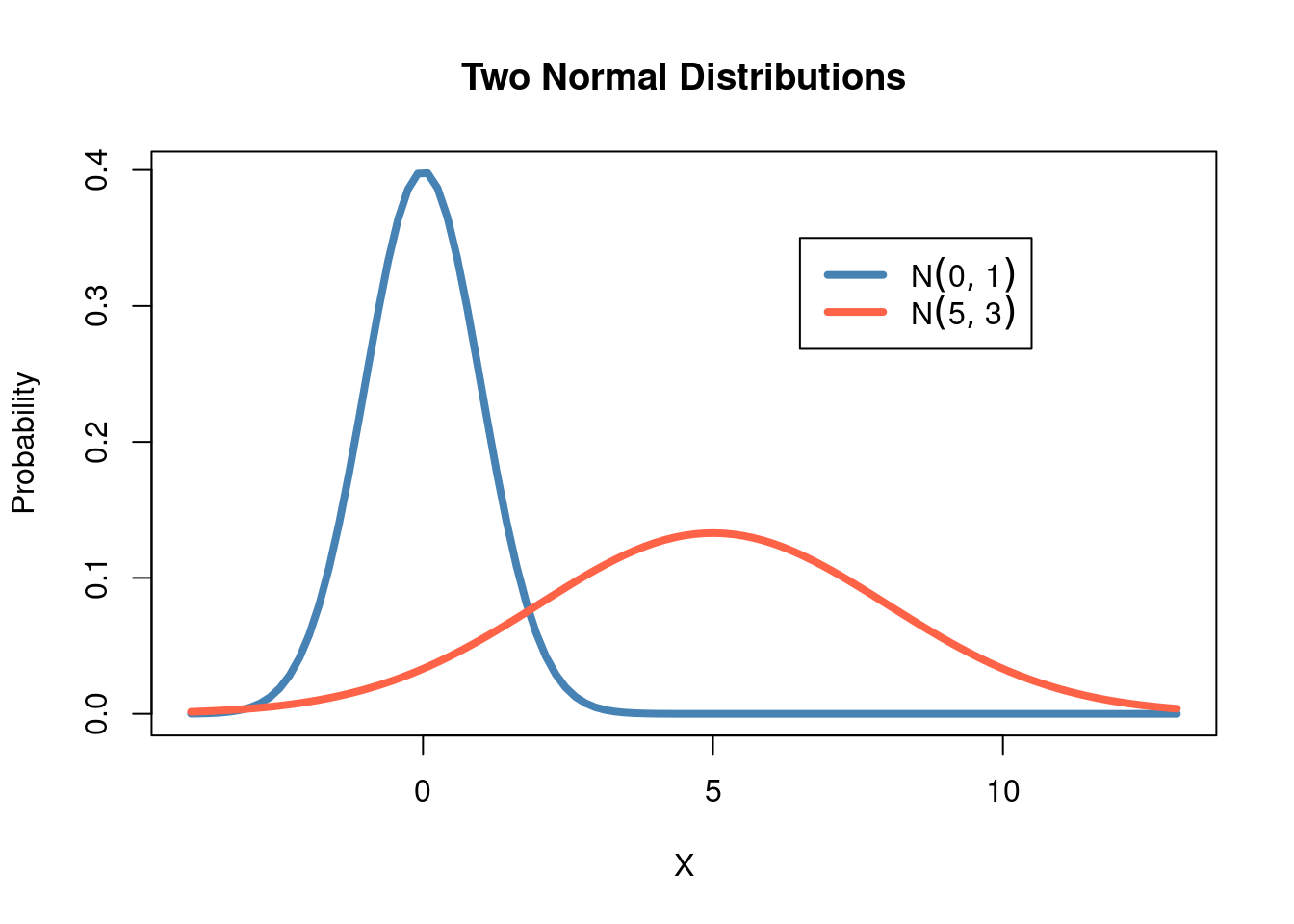Given that the normal distribution appears so frequently in statistics, it is common practice to standardize a normal distribution so that it has a mean value of $$\mu = 0$$ and variance $$\sigma^2 = 1$$ (note also that the standard deviation is $$\sigma = \sqrt{\sigma^2} = \sqrt{1} = 1$$). A normal random variable that has been standardized is called a standard normal distribution and is often written $$Z \sim N(0,1)$$. We can consider again the histograms above, once they’ve been standardized: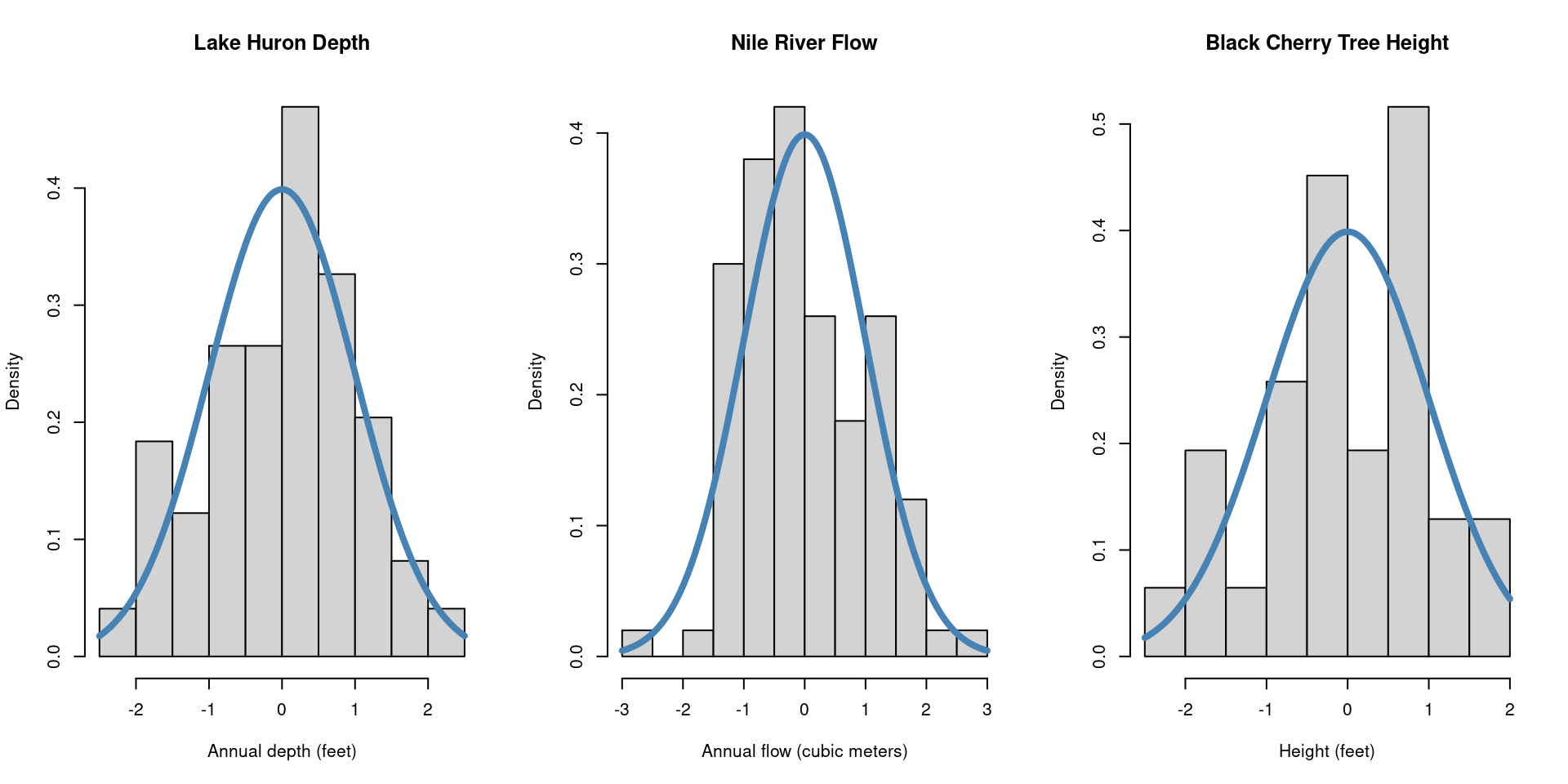Unlike the binomial distribution, in which there are $$n$$ possible values that our random variable can take, the normal distribution represents a random variable that is continuous over a range of values, making the normal distribution a member of the family of continuous distributions. Instead of asking the probability of a specific value, say, $$Z = 0$$, probabilities are given as the area under the curve for a certain interval. We might ask, “What is the probability that $$Z$$ is one standard deviation ($$\sigma$$) away from $$0$$?” or perhaps, “What is the probability that $$Z < 0 ?$$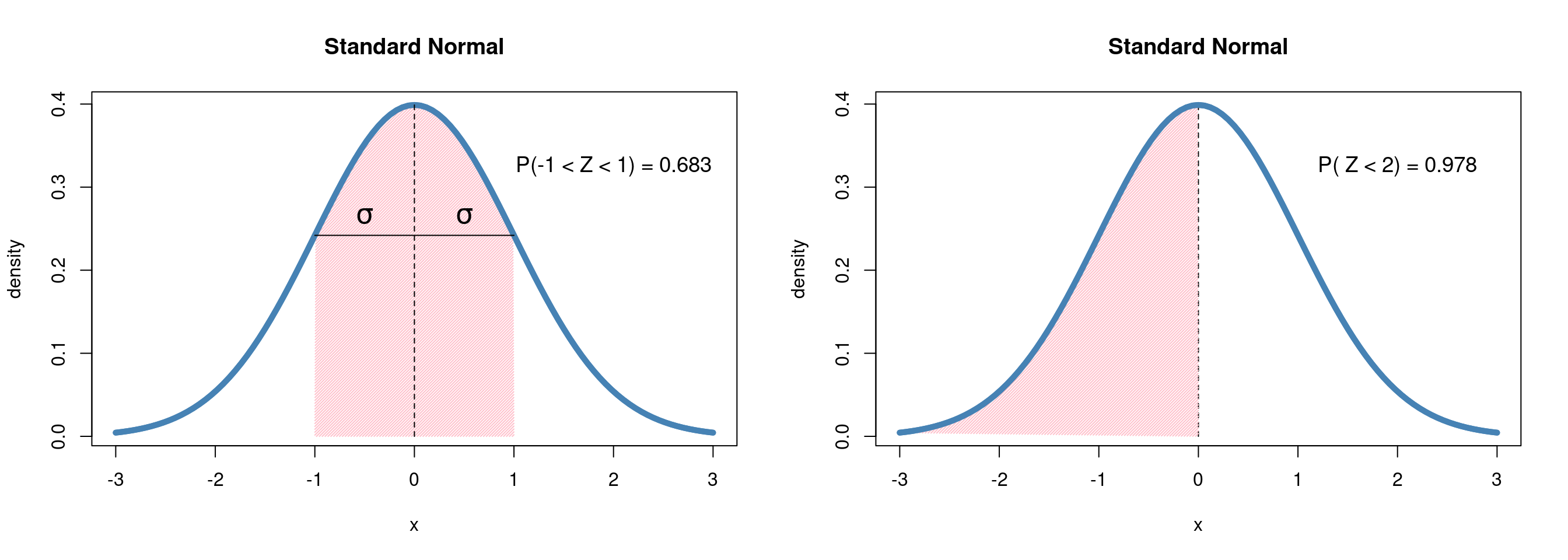We will return to the normal distribution in the following chapters, demonstrating both how it arises naturally in the study of statistics and the powerful toolkit it provides in the field of statistical inference.

Exercise 5.3

The applet below is designed to help you get familiar with the parameters of the normal distribution, and how they impact the probability distribution. You can change the values of the mean $$\mu$$, and the standard deviation $$\sigma$$, and the app will display the associated distribution in a probability histogram.

Use the applet to answer the following questions:

1. Start by setting $$\mu = 0$$ and $$\sigma = 1$$. How would you describe the shape of the distribution? Is it symmetric? How concentrated are the range of values around zero?

2. Now set $$\sigma = 2$$. What has changed? Reset the value back to $$\sigma = 1$$ and press the “Play” button. How does the normal distribution change with $$\sigma$$?

3. Repeat exercise 2, this time setting $$\sigma = 1$$ and allowing $$\mu$$ to change over a range of values. How does the distribution change? How does it change compared with what we saw in exercise 2?

4. Play around with different values of $$\mu$$ and $$\sigma$$. What impact does changing $$\mu$$ have on the concentration of values? How does changing $$\sigma$$ impact where the distribution is centered? How would you describe the relationship between $$\mu$$ and $$\sigma$$?

5. Compare your answers from this exercise with those from the binomial distribution. How do these distributions differ in their relationship to their parameters?

Exercise 5.4

The applet below is designed to familiarize you with data generated from the normal distribution. You can change the values of the mean $$\mu$$, and the standard deviation $$\sigma$$ to specify the parameters of the population distribution. Then, you can take a random sample from the distribution.

Use the applet to answer the following questions:

1. Start again by setting $$\mu = 0$$ and $$\sigma = 1$$, and simulate data several times with a sample size of 10. How does the observed data compare to the probability distribution? Does it have the same shape? What changes when we set our sample size to 50?

2. Compared with the binomial distribution, how well does data generated from a normal distribution tend to match the probability distribution? Given only the observed data, how close do you think your estimate would be of the underlying distribution?

Definition 5.3

Normal Distribution: A continuous bell-shaped distribution with two parameters that are the mean value, $$\mu$$, with a variance $$\sigma^2$$

Standard Normal Distribution: A special case of the normal distribution, $$Z \sim N(0, 1)$$

Continuous Distribution: Any probability distribution that depicts the occurrence of a random variable that can take an infinite set of possible values within a given (potentially infinite) interval